## 前言

• 数据：data
• 统计变换：stats
• 几何对象：geom
• 图形属性：aes
• 标尺：scale
• 图层：layer
• 坐标系：coord
• 分面：facet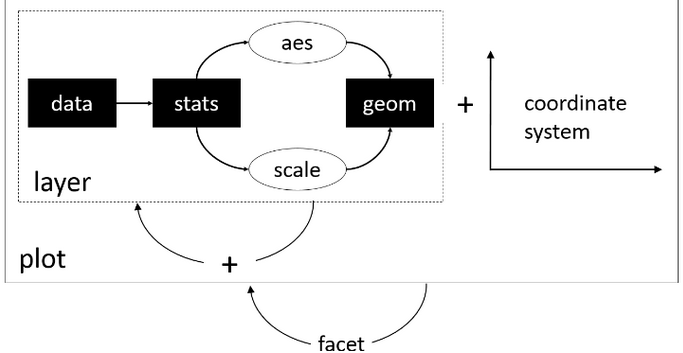• 标准化：任何一个统计图形遵循相同的绘图流程，所以语法高度统一；
• 面向数据：上面的绘图流程只与数据有关，与数据无关的绘图细节封装在单独的theme()方法里，数据相关绘图与数据无关绘图分离；

## 数据

ggplot2接受的输入数据一般是data.frame，这是一个表格型结构，每一行是一个观测（observation），每一列是一个变量（variable）。R语言内置了许多著名的数据集，本文选取其中的iris进行讲解。iris中文名是鸢尾花，有四个属性，分别是Sepal.Length（花萼长度），Sepal.Width（花萼宽度），Petal.Length（花瓣长度），Petal.Width（花瓣宽度），以及一个类别标签Species。我在网上找了一个图片，做个标注，方便朋友理解。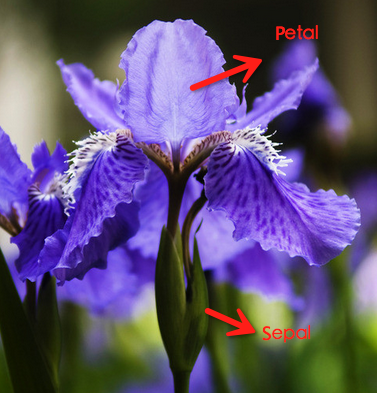str(iris)
## 'data.frame':    150 obs. of  5 variables:
##  $Sepal.Length: num 5.1 4.9 4.7 4.6 5 5.4 4.6 5 4.4 4.9 ... ##$ Sepal.Width : num  3.5 3 3.2 3.1 3.6 3.9 3.4 3.4 2.9 3.1 ...
##  $Petal.Length: num 1.4 1.4 1.3 1.5 1.4 1.7 1.4 1.5 1.4 1.5 ... ##$ Petal.Width : num  0.2 0.2 0.2 0.2 0.2 0.4 0.3 0.2 0.2 0.1 ...
##  $Species : Factor w/ 3 levels "setosa","versicolor",..: 1 1 1 1 1 1 1 1 1 1 ... summary(iris) ## Sepal.Length Sepal.Width Petal.Length Petal.Width ## Min. :4.300 Min. :2.000 Min. :1.000 Min. :0.100 ## 1st Qu.:5.100 1st Qu.:2.800 1st Qu.:1.600 1st Qu.:0.300 ## Median :5.800 Median :3.000 Median :4.350 Median :1.300 ## Mean :5.843 Mean :3.057 Mean :3.758 Mean :1.199 ## 3rd Qu.:6.400 3rd Qu.:3.300 3rd Qu.:5.100 3rd Qu.:1.800 ## Max. :7.900 Max. :4.400 Max. :6.900 Max. :2.500 ## Species ## setosa :50 ## versicolor:50 ## virginica :50 ## ## ##  Hadley对data.frame提出了一个是否tidy的概念，抽象来讲就是一个变量必须有自己独立的一列，一个观测必须有自己独立的一行，每个取值必须有自己独立的一个单元格。为了便于理解，我们从R for Data Science这本书截取出这个图进行解释：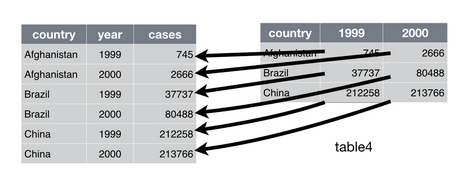左边的数据是tidy的，右边的数据是不tidy的，通过另一个包tidyr可以轻松完成二者的转换。ggplot2的数据要求是tidy的。 ## 几何对象、图形属性、图层 ### 几何对象 几何对象，说的直观一些，就是你要画什么图来表示这组数据。ggplot2提供了众多几何对象geom_xyz()供大家选择。举两个常见的例子，geom_point()用于表示两个连续变量之间的关系，几何形状是点；geom_bar()用于表示x轴为离散变量，y轴为连续连续变量之间的关系，几何形状是条块。完整的几何对象请下载RStudio公司总结的ggplot2 cheetsheet 几何对象有一个position选项，用于指定如何在空间内布置相同取值的集合对象。dodge为并排模式；fill为堆叠模式，并归一化为相同的高度；stack为纯粹的堆叠模式；jitter会在X和Y两个方向增加随机的扰动来防止对象之间的覆盖。 ### 图形属性 每个几何对象都有自己的属性，这些属性的取值需要通过数据提供。数据与图形属性之间的映射关系称为mapping，在ggplot2中用aes()进行定义。常见的图形属性有：xysizecolorgroup。图形属性的任意一项都可以用数据的某一个变量来表示。 ### 图层 ggplot2的绘图过程有点像Photoshop，有一个图层的理念，每个图层可以有自己的图形对象和图形属性，通过+将不同图层叠加起来生成最后的统计图形。如果将数据定义在ggplot()中，那么所有图层都可以共用这个数据；如果将数据定义在geom_xyz()中，那么这个数据就只供这个几何对象使用。 掌握了数据、几何对象、图形属性和图层的概念后，我们就可以开始绘制常见的统计图形了。 ### 基本绘图 Kaggle数据挖掘竞赛里有一个经典的探索性分析例子，对iris数据集进行了各种形式的可视化，帮助人通过直观的图形更深地理解特征与label的关系。Kaggle官网给出了Python版本的实现。本节用R对该notebook的代码进行重现。 library(ggplot2) # Make scatter plot of Sepal.Length and Sepal.Width p.scatter <- ggplot(iris) + geom_point(aes(x=Sepal.Length, y=Sepal.Width)) p.scatter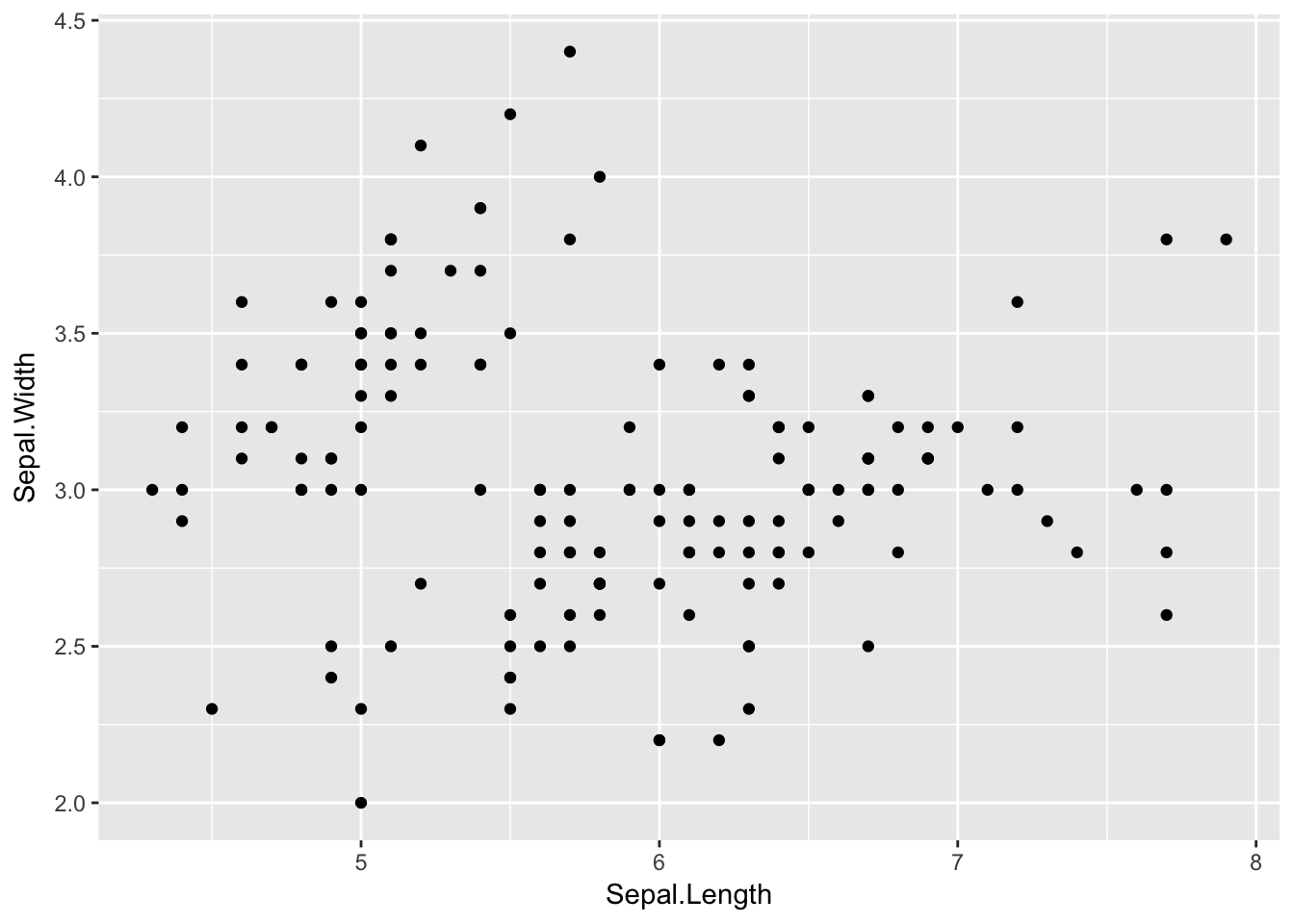# One piece of information missing in the plots above is what species each plant is p.scatter <- ggplot(iris) + geom_point(aes(x=Sepal.Length, y=Sepal.Width, color=Species)) p.scatter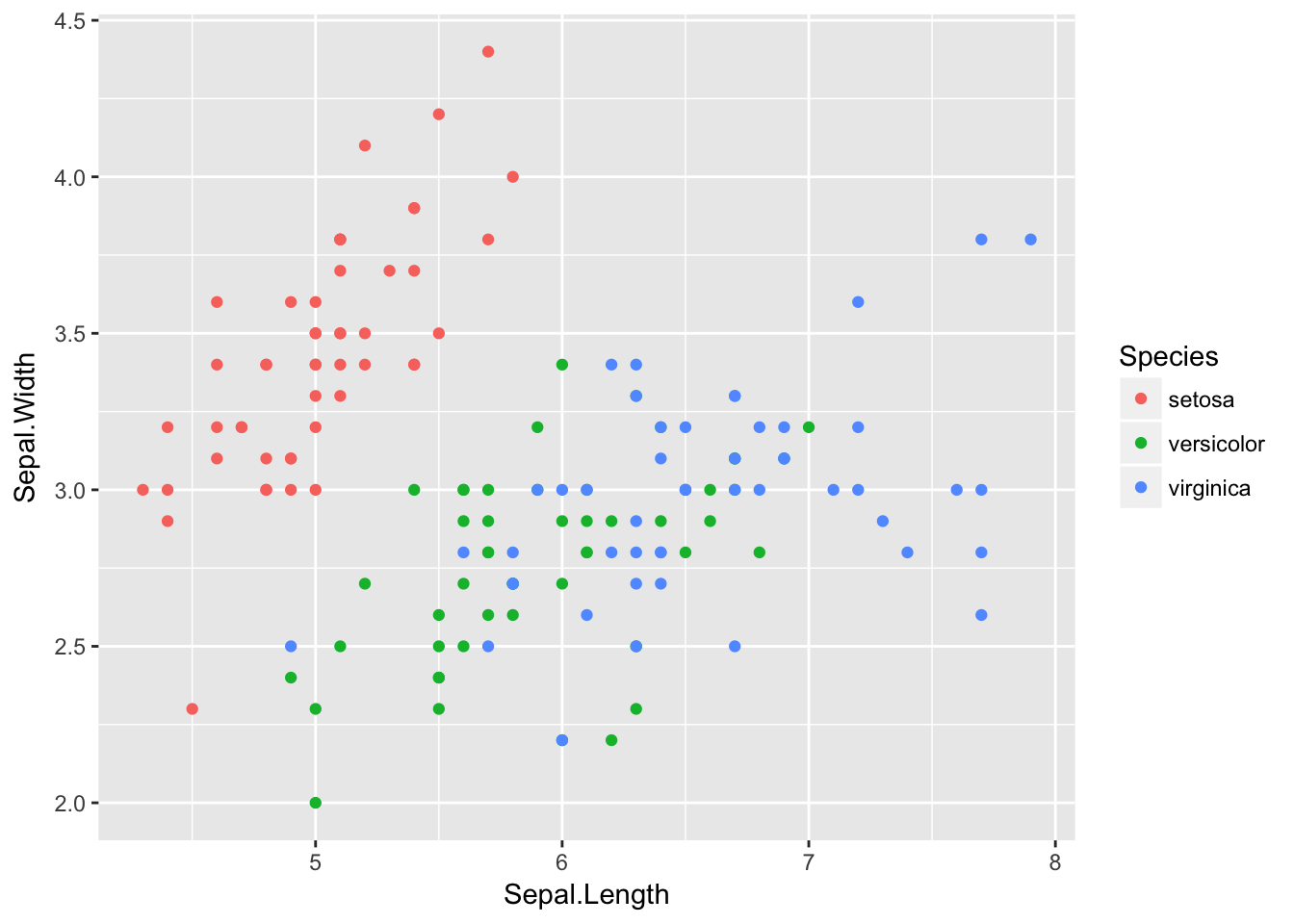# Boxplot to explore numeric variable p.box <- ggplot(iris) + geom_boxplot(aes(x=Species, y=Petal.Length)) p.box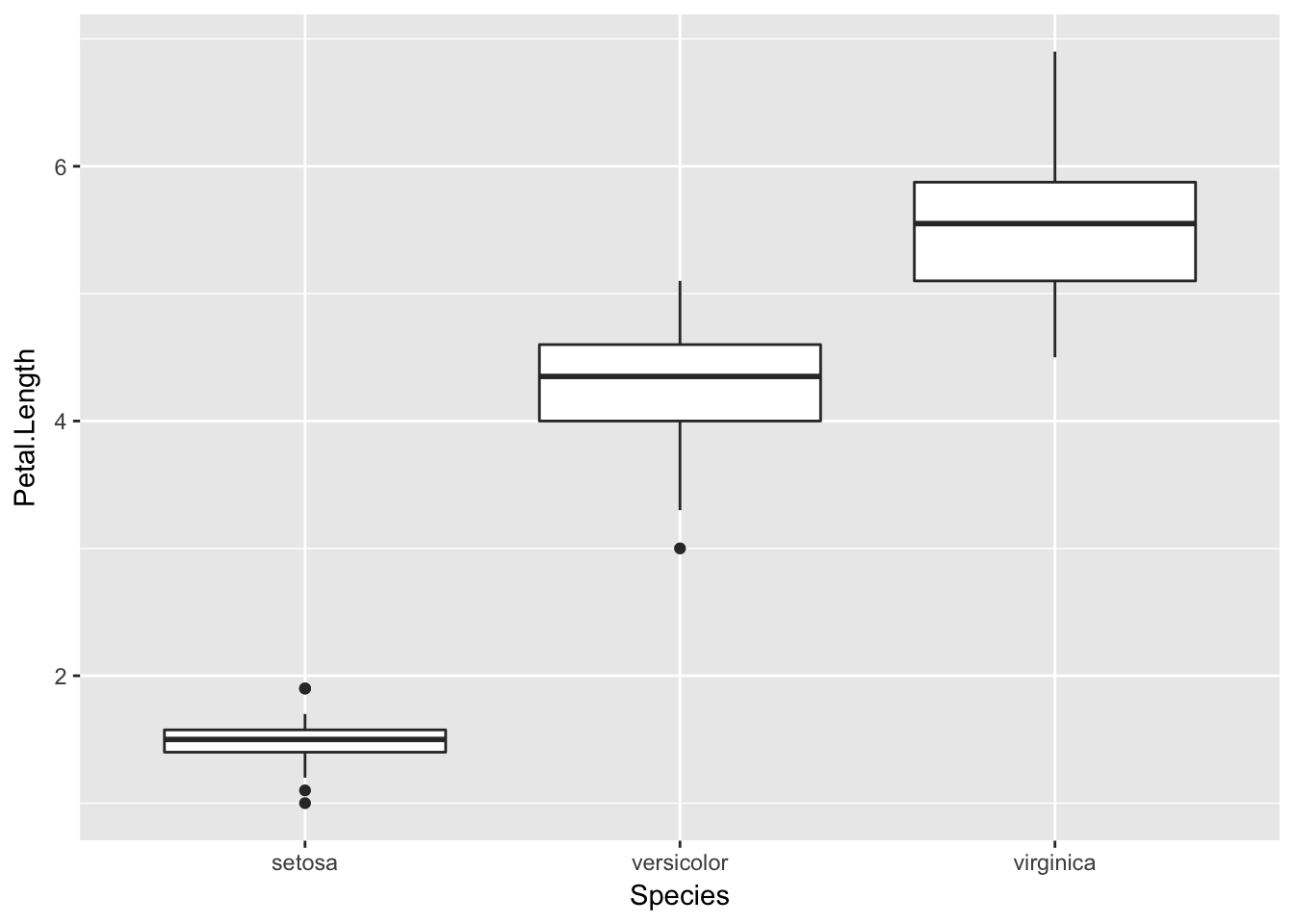# One way we can extend this plot is adding a layer of individual points on top of it p.box.jitter <- p.box + geom_jitter(aes(x=Species, y=Petal.Length)) p.box.jitter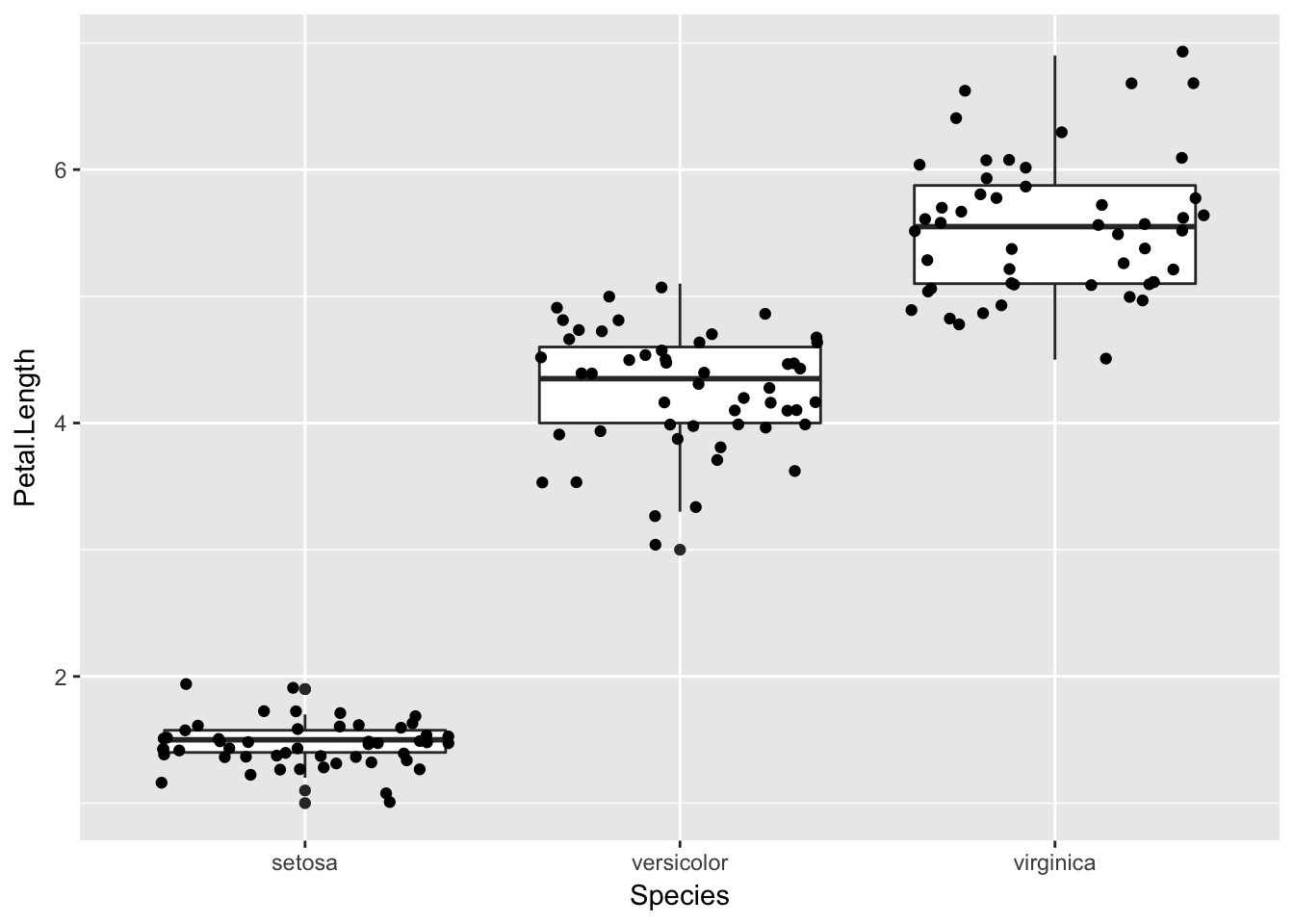# A violin plot combines the benefits of the previous two plots and simplifies them # Denser regions of the data are fatter, and sparser thiner in a violin plot p.violin <- ggplot(iris) + geom_violin(aes(x=Species, y=Petal.Length)) p.violin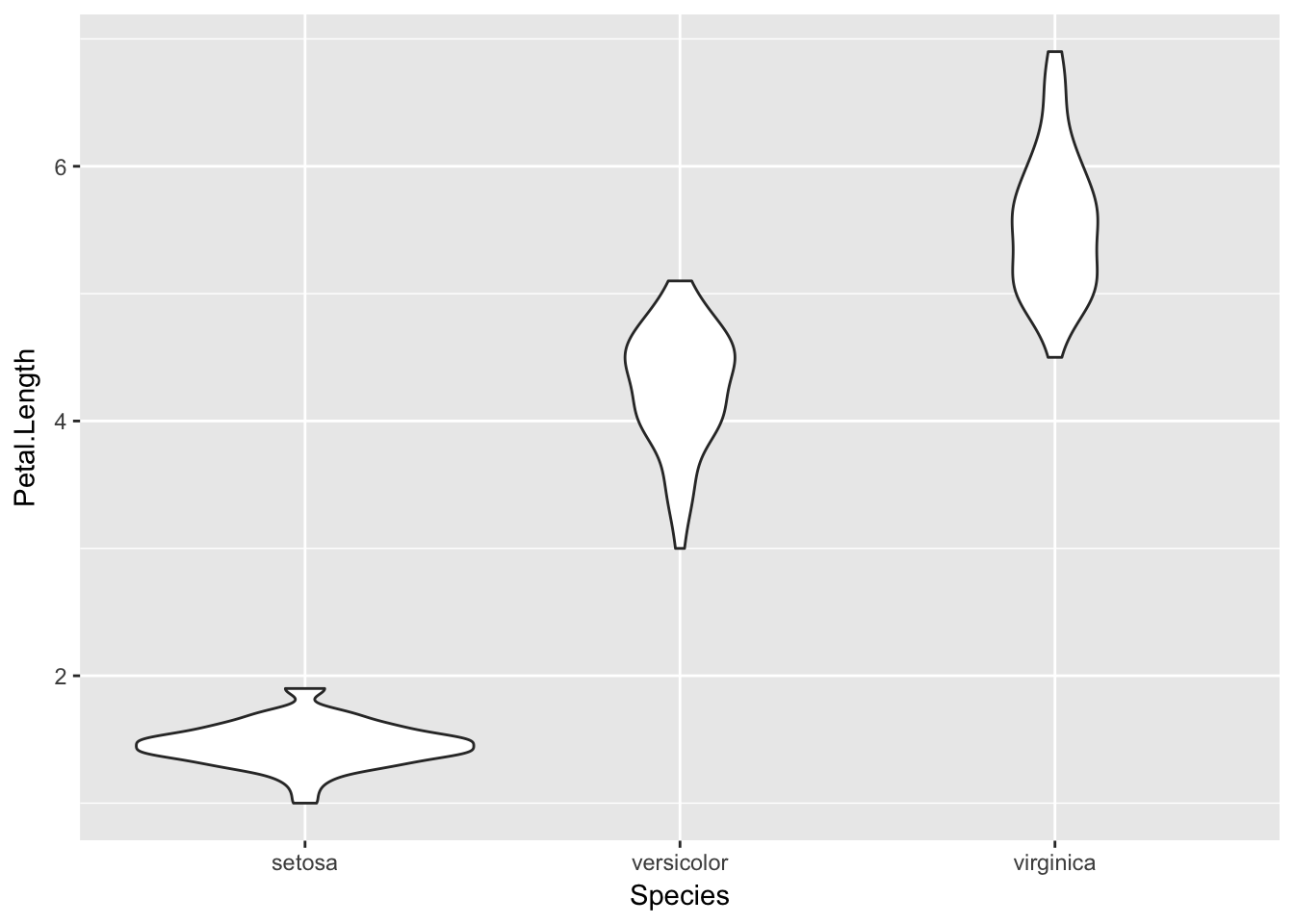# A final plot useful for looking at univariate relations is the kdeplot, p.density <- ggplot(iris) + geom_density(aes(x=Petal.Length, colour=Species)) p.density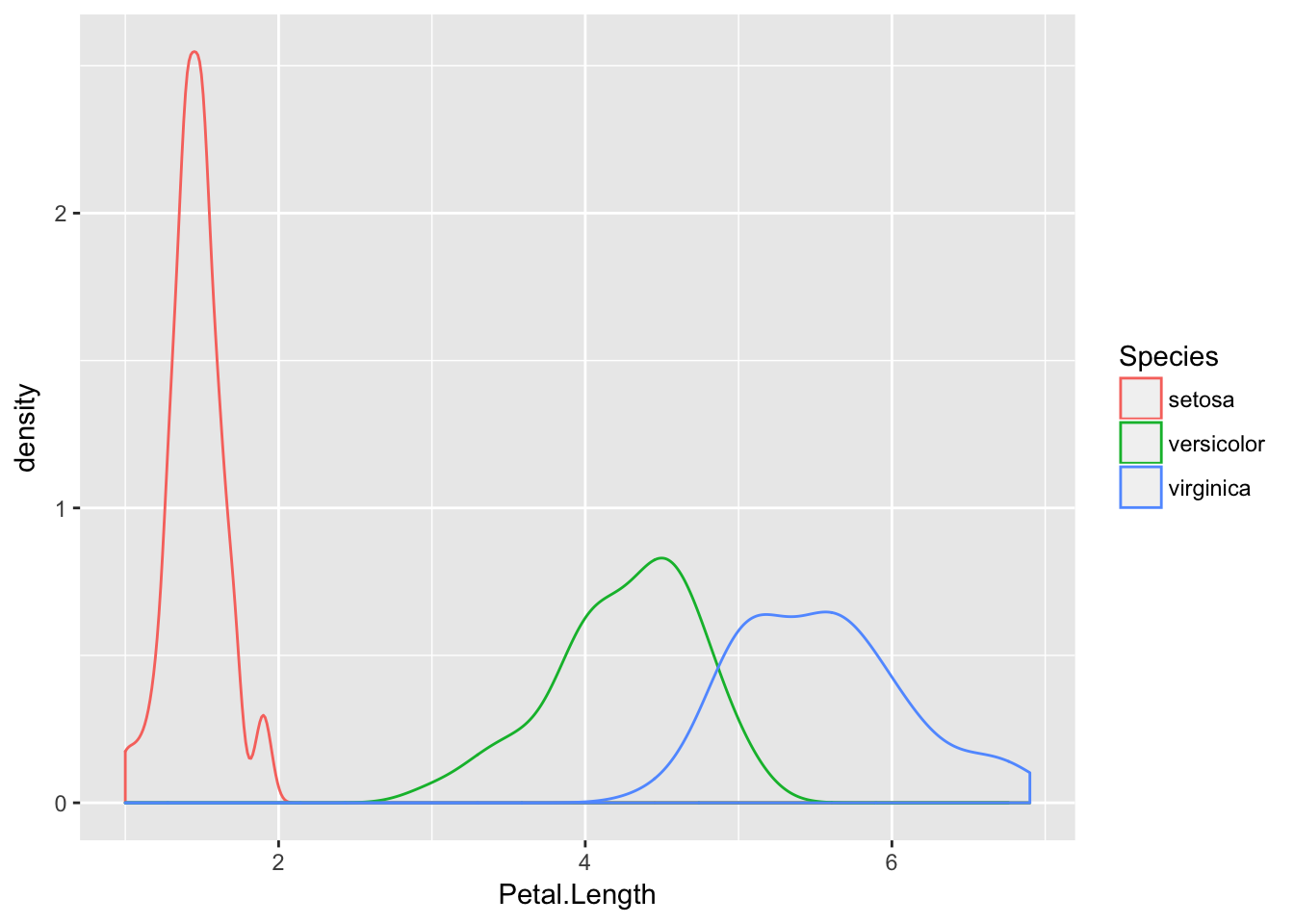## 分面与布局 分面，就是分组绘图，根据定义的规则，将数据分为多个子集，每个子集按照统一的规则单独制图，排布在一个页面上。ggplot2提供两种分面模式：facet_grid()facet_wrap() 我们先来看一下facet_grid()的效果。 library(tidyr) library(dplyr) # 将数据变为tidy的 tidy_iris <- iris %>% gather(feature_name, feature_value, one_of(c("Sepal.Length", "Sepal.Width", "Petal.Length", "Petal.Width"))) p.box.facet <- ggplot(tidy_iris) + geom_boxplot(aes(x=Species, y=feature_value)) + facet_grid(feature_name~Species) p.box.facet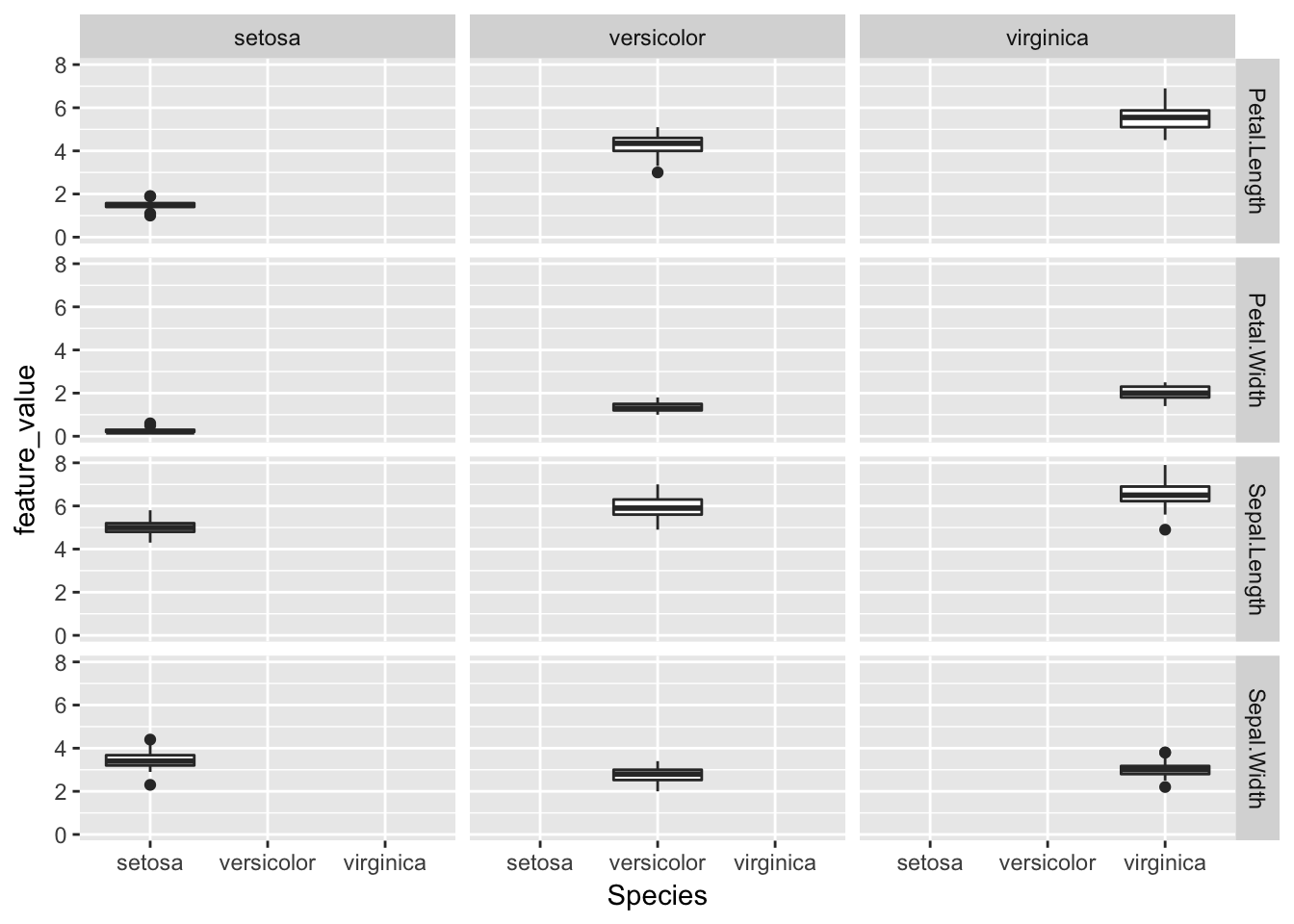可以看到facet_grid()是一个二维的矩形布局，每个子集的位置由行位置变量~列位置变量的决定，在上面的例子中就是每一个Species的取值作为一行，每一个feature_name的取值作为一列。 再来看一下facet_wrap()的效果。 p.box.facet <- ggplot(tidy_iris) + geom_boxplot(aes(x=Species, y=feature_value)) + facet_wrap(~feature_name+Species, scales="free") p.box.facet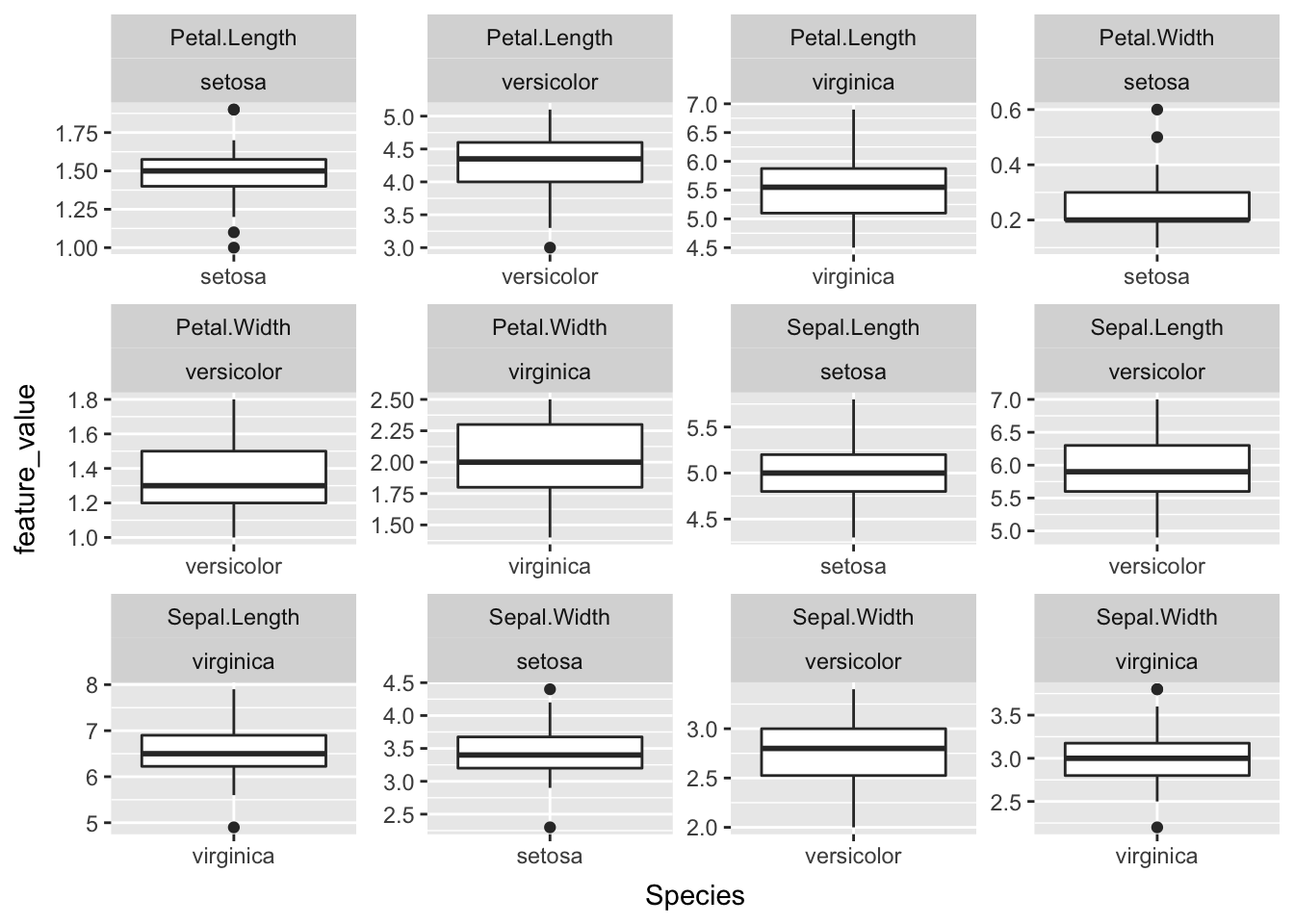facet_wrap()生成一个动态调整的一维布局，根据~位置变量1+位置变量2+...来确定每个子集的位置，先逐行排列，放不下了移动到下一行。scales="free"让每个子图的坐标系适合自己的数据，便于在有限的空间里充分展示子图的细节，但也失去了不同子图之间比较的作用，需要谨慎使用。 分面的特点是可以快速生成多个子图，每个子图的生成方式是一样的，因此只需要指定分组的规则即可。但是有时候我们希望绘制多个子图，每个子图的生成方法却不一样，这个时候分面就不起作用了，需要使用grid包提供的布局功能。下面我们用ggplot2和grid的布局实现一个较为复杂的统计图形效果： library(grid) # Show bivariate scatter plot and univariate histogram p.hist.len <- ggplot(iris) + geom_histogram(aes(x=Sepal.Length)) p.hist.wid <- ggplot(iris) + geom_histogram(aes(x=Sepal.Width)) + coord_flip() grid.newpage() pushViewport(viewport(layout = grid.layout(3, 3))) print(p.scatter, vp=viewport(layout.pos.row=2:3, layout.pos.col=1:2)) print(p.hist.len, vp=viewport(layout.pos.row=1, layout.pos.col=1:2)) print(p.hist.wid, vp=viewport(layout.pos.row=2:3, layout.pos.col=3))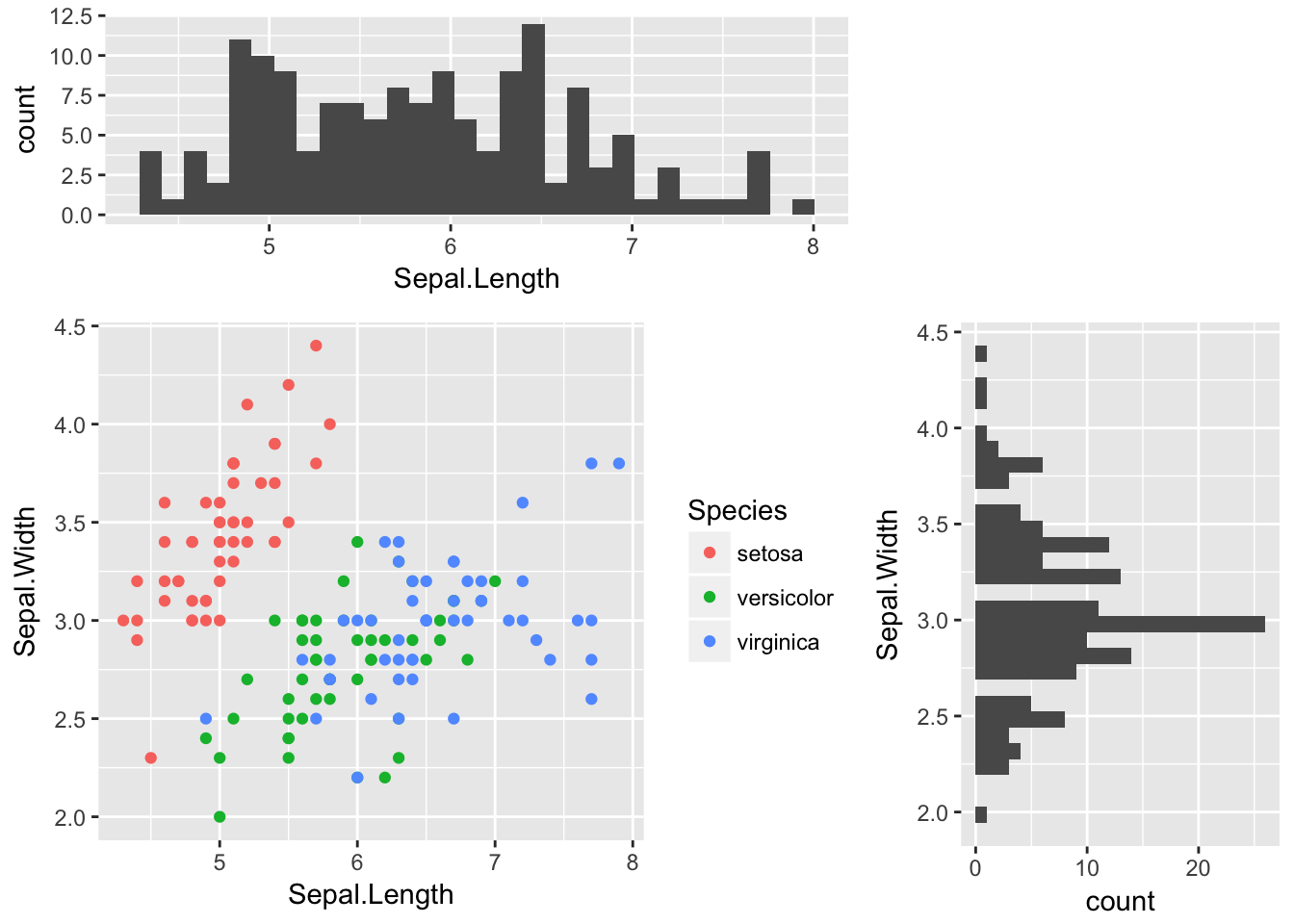在做数据分析时，我们经常需要观察变量自身与变量之间的两两关系。这个过程中需要绘制大量的图表，且每个业务的数据分析都需要这么做，因此算是一种重复性比较大的工作。我们可以使用GGally包来快速完成这个探索性分析的任务。 library(GGally) # Another useful seaborn plot is the pairplot, which shows the bivariate relation # between each pair of features # # From the pairplot, we'll see that the Iris-setosa species is separataed from the other # two across all feature combinations ggpairs(iris, aes(colour=Species), alpha=0.4) # R could be better!!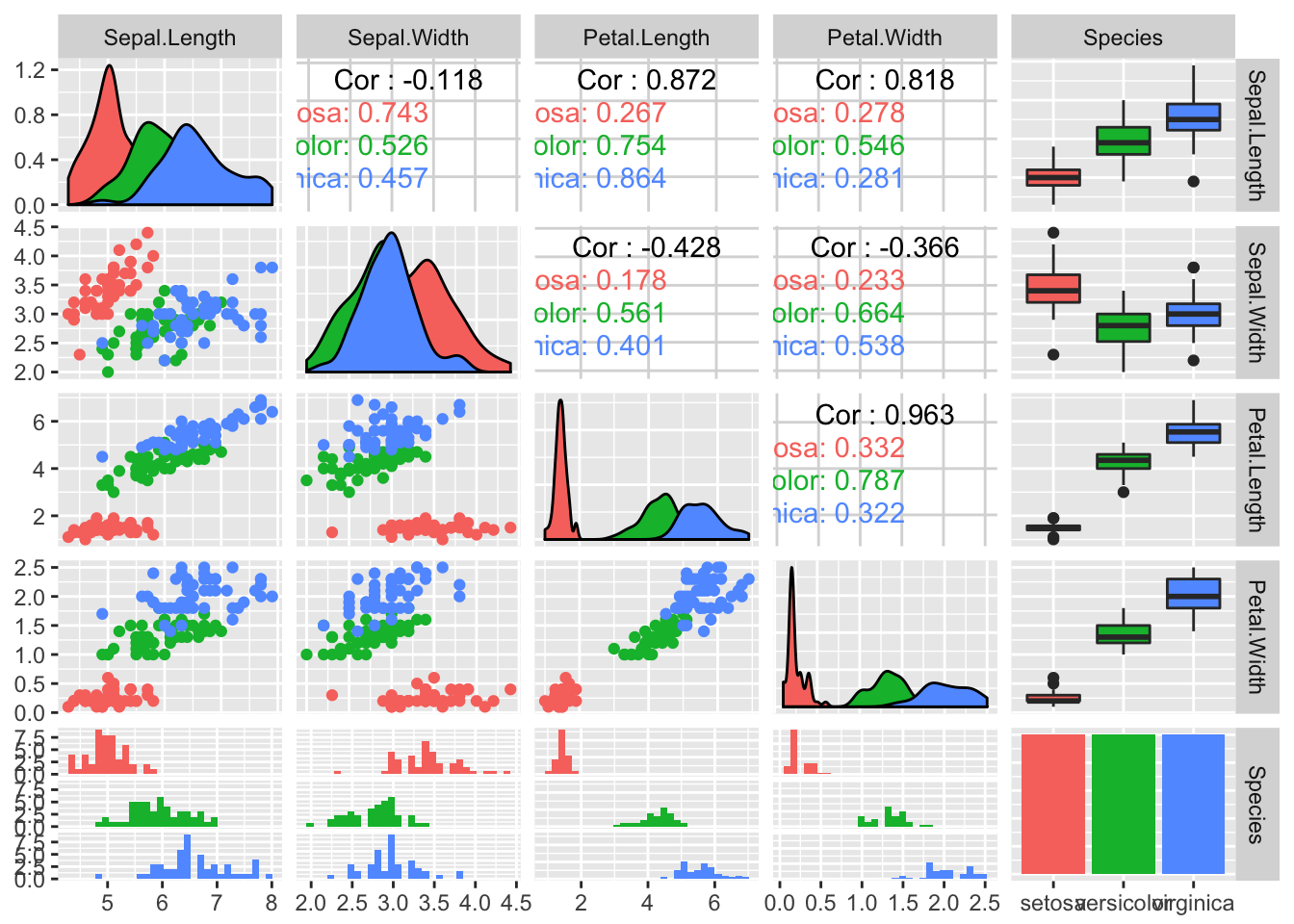Kaggle数据挖掘竞赛剩下的例子是绘制Parallel coordinate graph、Andrews Curve、radviz，前两个的实现参考如下，最后一个暂时没找到对应的方法。 # Parallel coordinate graph & Andrews Curve # 修改自：http://cos.name/2009/03/parallel-coordinates-and-andrews-curve/ # 轮廓图的思想非常简单、直观，它是在横坐标上取n个点，依次表示各个指标(即变量)；横坐标上则对应各个指标的值(或者经过标准化变换后的值)，然后将每一组数据对应的点依次连接即可 # 调和曲线图的思想和傅立叶变换十分相似： # 根据三角变换方法将 n 维空间的点映射到二维平面上的曲线上，其中x取值范围为[-pi,pi]。 # Another multivariate visualization technique pandas has is parallel_coordinates # Parallel coordinates plots each feature on a separate column & then draws lines # connecting the features for each data sample p.paral <- ggplot(cbind(iris %>% gather(feature_name, feature_value, one_of(c("Sepal.Length", "Sepal.Width", "Petal.Length", "Petal.Width"))), id=1:nrow(iris))) + geom_line(aes(x=feature_name, y=feature_value, group=id, colour=Species)) p.paral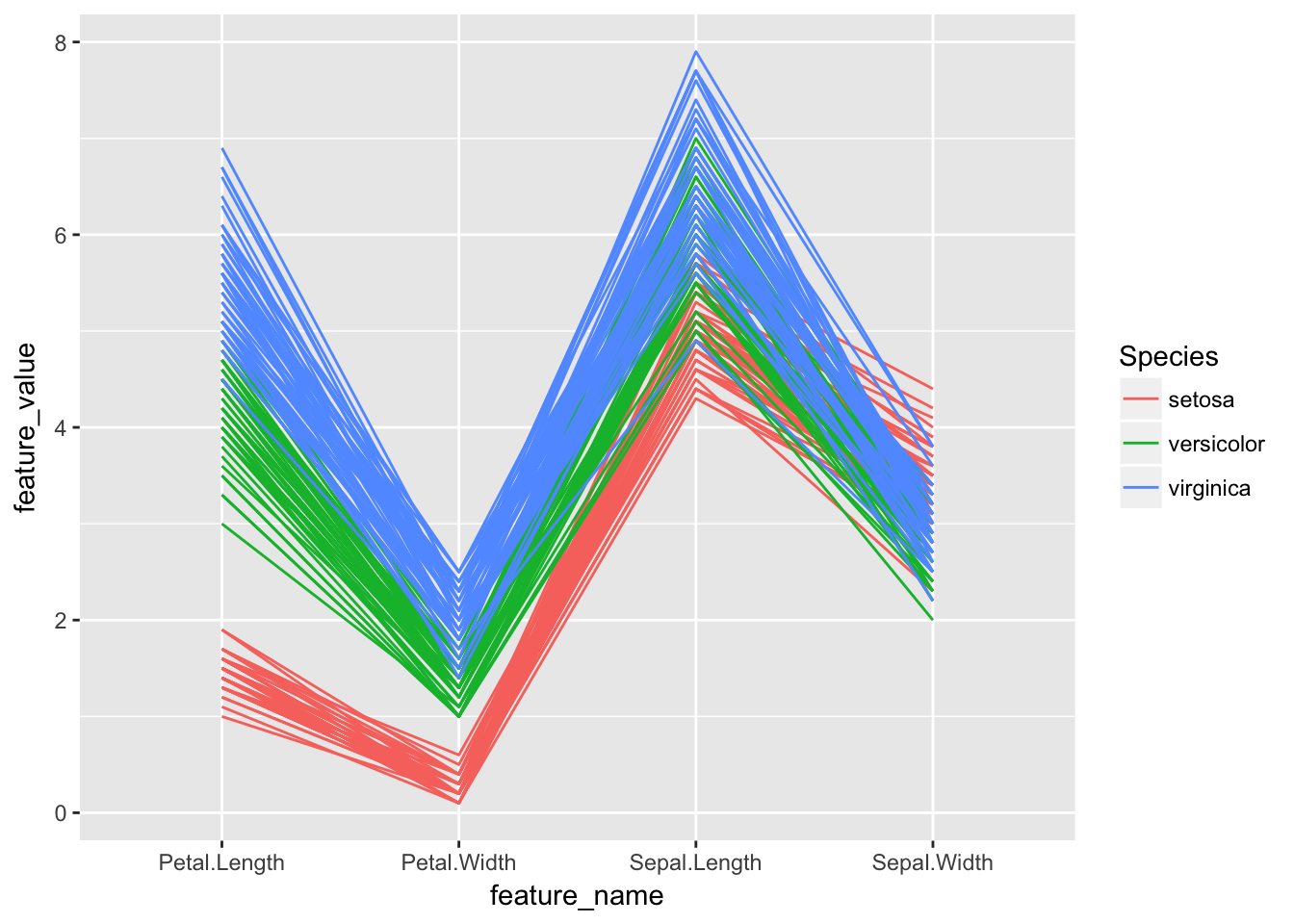# One cool more sophisticated technique pandas has available is called Andrews Curves # Andrews Curves involve using attributes of samples as coefficients for Fourier series # and then plotting these andrews_curve <- function(data, x_col, y_col, step=pi/30){ x = as.matrix(data[, x_col]) t = seq(-pi, pi, pi/30) m = nrow(x) n = ncol(x) f = matrix(0, m, length(t)) for(i in 1:m) { f[i,] = x[i,1]/sqrt(2) for(j in 2:n) { if (j%%2 == 0) f[i, ] = f[i, ] + x[i, j] * sin(j/2 * t) else f[i, ] = f[i, ] + x[i, j] * cos(j%/%2 * t) } } colnames(f) <- t label <- data[, y_col] id <- c(1:nrow(f)) res <- cbind(as.data.frame(f), label, id) %>% gather(x, y, -label, -id, convert = TRUE) } iris.andrew <- andrews_curve(iris, x_col=c("Sepal.Length", "Sepal.Width", "Petal.Length", "Petal.Width"), y_col="Species") p.andrew <- ggplot(iris.andrew) + geom_line(aes(x, y, group=id, color=label)) p.andrew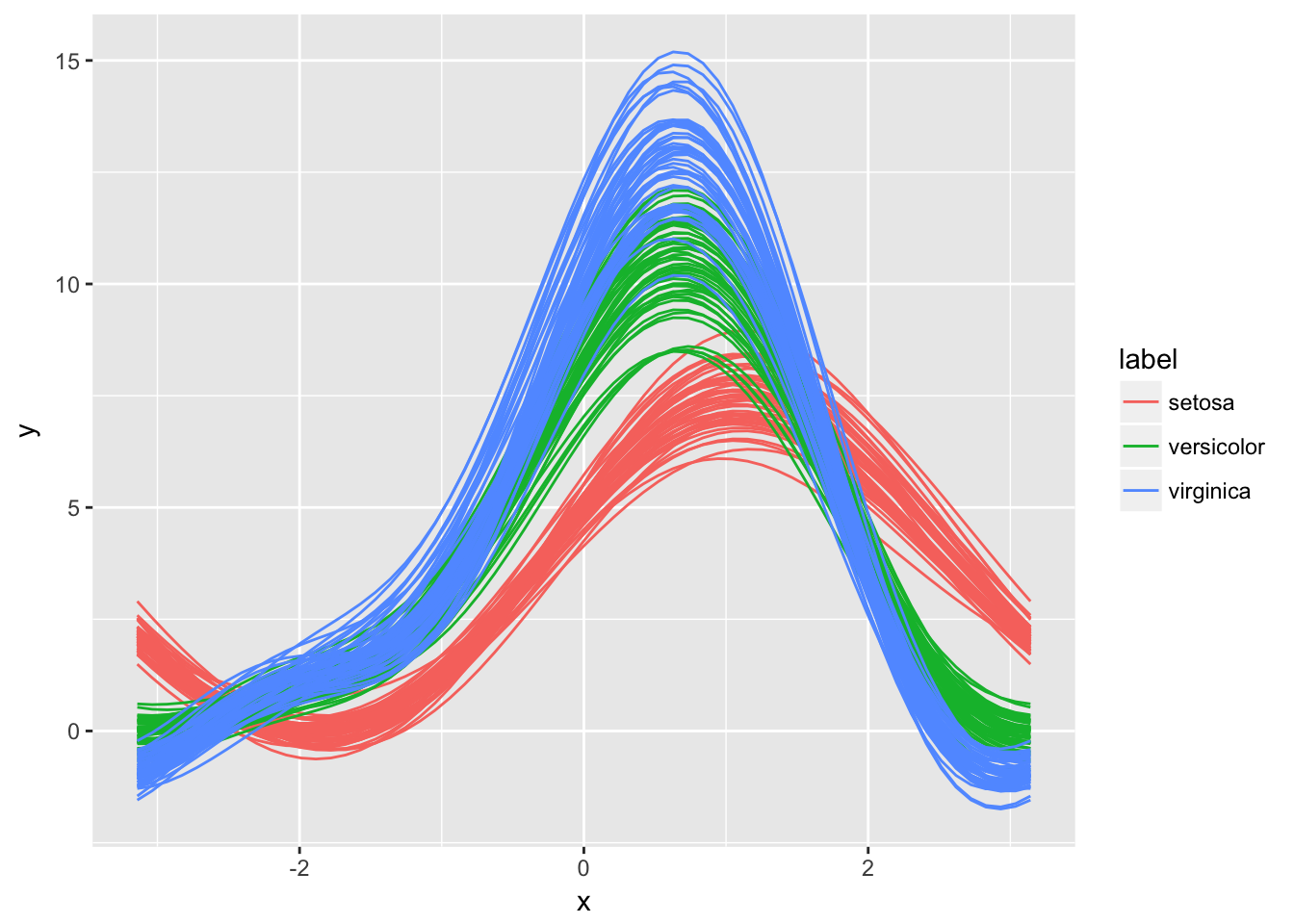# A final multivariate visualization technique pandas has is radviz # Which puts each feature as a point on a 2D plane, and then simulates # having each sample attached to those points through a spring weighted # by the relative value for that feature # 暂时没能力实现 ## 标尺 前面提到aes()设定了数据与图形属性的映射关系，但是数据怎么映射为属性，这就是标尺（Scales）的功能。对于任何一个图形属性，如xyalphacolorfilllinetypeshapesize，ggplot2都提供以下四种标尺： • scale_*_continuous()：将数据的连续取值映射为图形属性的取值 • scale_*_discrete()：将数据的离散取值映射为图形属性的取值 • scale_*_identity()：使用数据的值作为图形属性的取值 • scale_*_mannual()：将数据的离散取值作为手工指定的图形属性的取值 举个例子 group_iris <- iris %>% group_by(Species) %>% dplyr::summarise(avg_sepal_length=mean(Sepal.Length)) str(group_iris) ## Classes 'tbl_df', 'tbl' and 'data.frame': 3 obs. of 2 variables: ##$ Species         : Factor w/ 3 levels "setosa","versicolor",..: 1 2 3
##  \$ avg_sepal_length: num  5.01 5.94 6.59
p <- ggplot(group_iris) + geom_bar(aes(x=Species, weight=avg_sepal_length, fill=Species))
p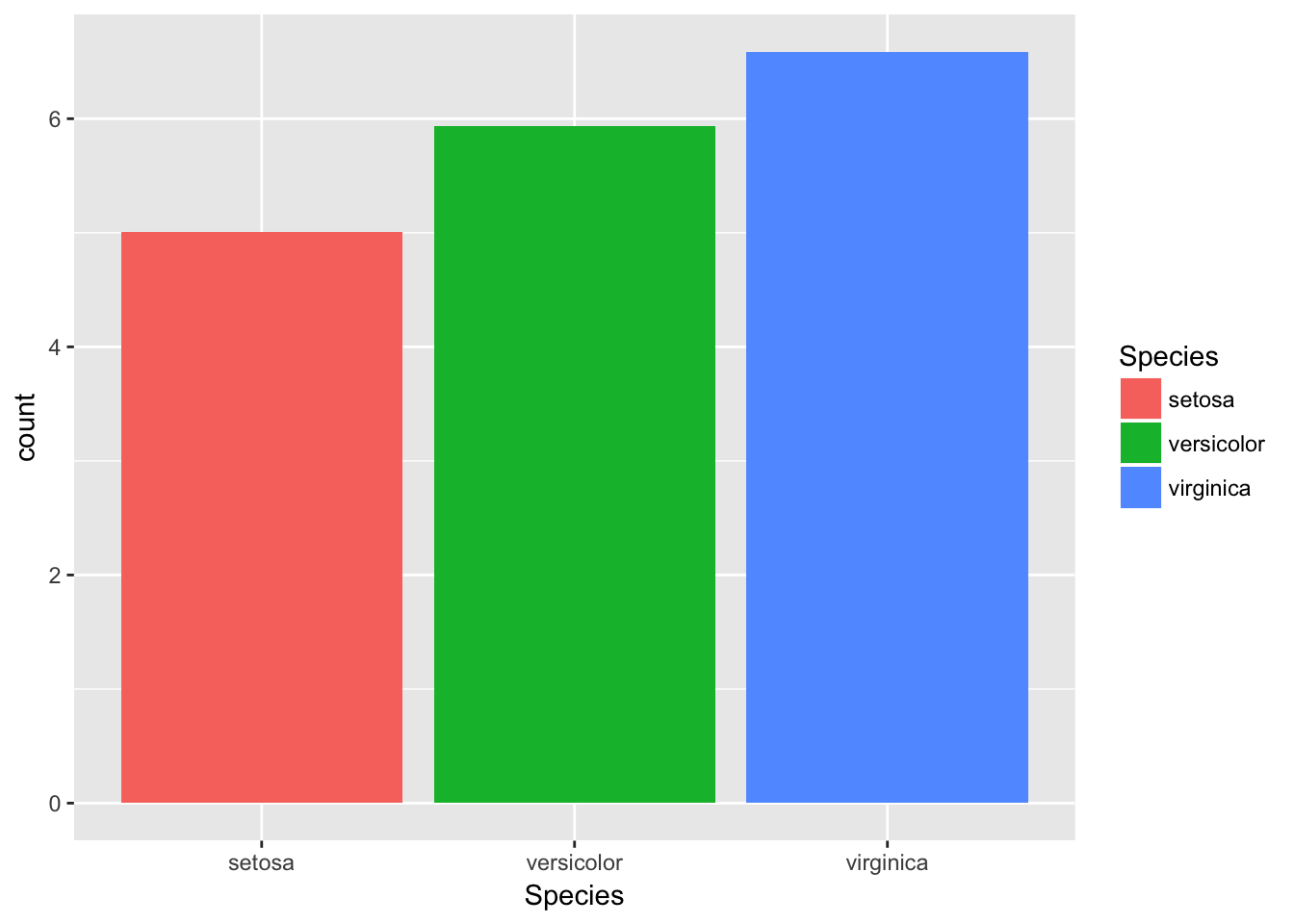p + scale_fill_manual(
values = c("skyblue", "royalblue", "navy"), # mannual类scale特有的选项，指定图形属性的取值范围
limits = c("setosa", "versicolor", "virginica"), # 数据的取值范围
breaks = c("setosa", "versicolor", "virginica"), # 图例和轴要显示的分段点
name = "Species",  # 图例和轴使用的名称
labels = c("set", "ver", "vir")  # 图例使用的标签
)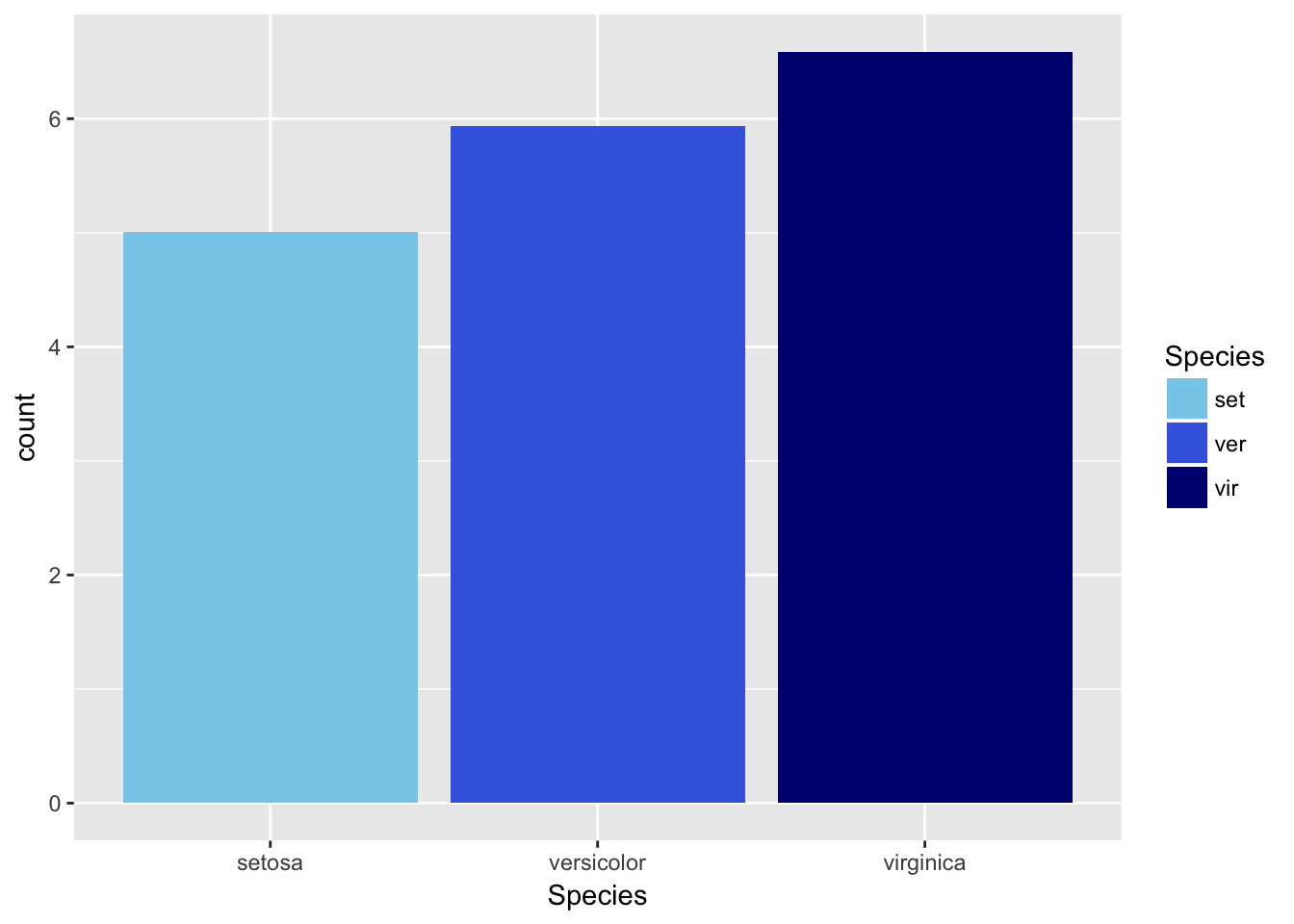• scale_x_date(labels=date_format("%m/%d"), breaks=date_breaks("2 weeks"))
• scale_x_datetime()
• scale_x_log10()
• scale_x_reverse()
• scale_x_sqrt()

• scale_fill_brewer(palette="Blues")：根据调色盘生成颜色标尺，可用的调色盘可以通过RColorBrewer::display.brewer.all()命令查看；对于具体的一个调色盘，可以通过RColorBrewer::brewer.pal(n=4, name="Blues")查看具体某个名字调色盘的n个配色值。
• scale_fill_grey(start=0.2, end=0.8, na.value=“red”)：灰度标尺
• scale_fill_gradient(low="red", high="yellow")：双色渐变标尺
• scale_fill_gradient2(low="red", high="blue", mid="white", midpoint=25)：三色渐变标尺
• scale_fill_gradientn(colours=terrain.colors(6))：n色渐标尺，其他的调色盘有rainbow()heat.colors()topo.colors()cm.colors()以及RColorBrewer包的调色盘。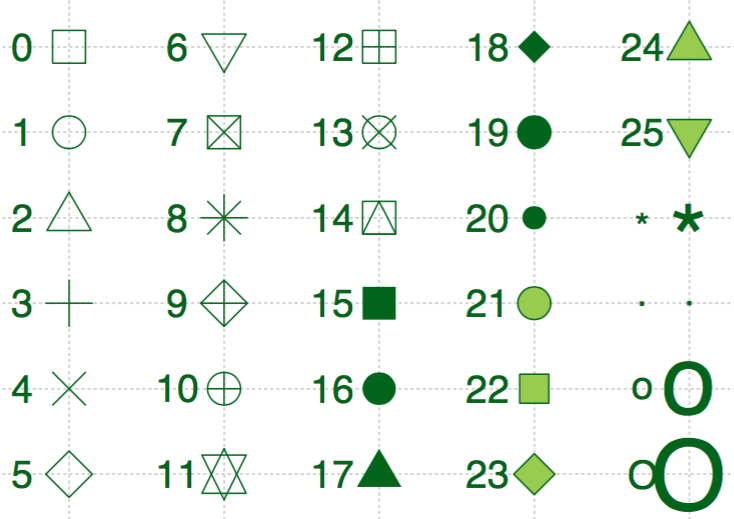## 坐标系

ggplot2默认的坐标系是笛卡尔坐标系，可以用如下方法指定取值范围：coord_cartesian(xlim=c(0,5), ylim=c(0,3))。如果想要让x轴和y轴换位置，比如将柱形图换成条形图，可以使用coord_flip()函数。coord_polar(theta="x", direction=1)是角度坐标系,theta指定角度对应的变量，start指定起点离12点钟方向的偏离值，direction若为1表示顺时针方向，若为-1表示逆时针方向。

## 图例

ggplot2可以设定图例的位置：theme(legend.position="bottom")，其他选项有top、left和right。

## 标签

• ggtitle("New Plot Title")：指定图形名称
• xlab("New X label")：指定x轴标签
• ylab("New Y label")：指定y轴标签
• 图例标签需要使用scale_*()namelabels`选项进行指定